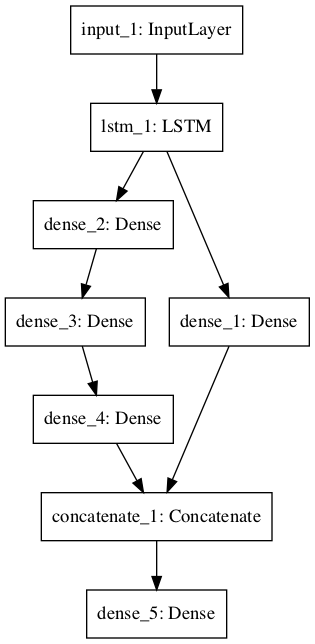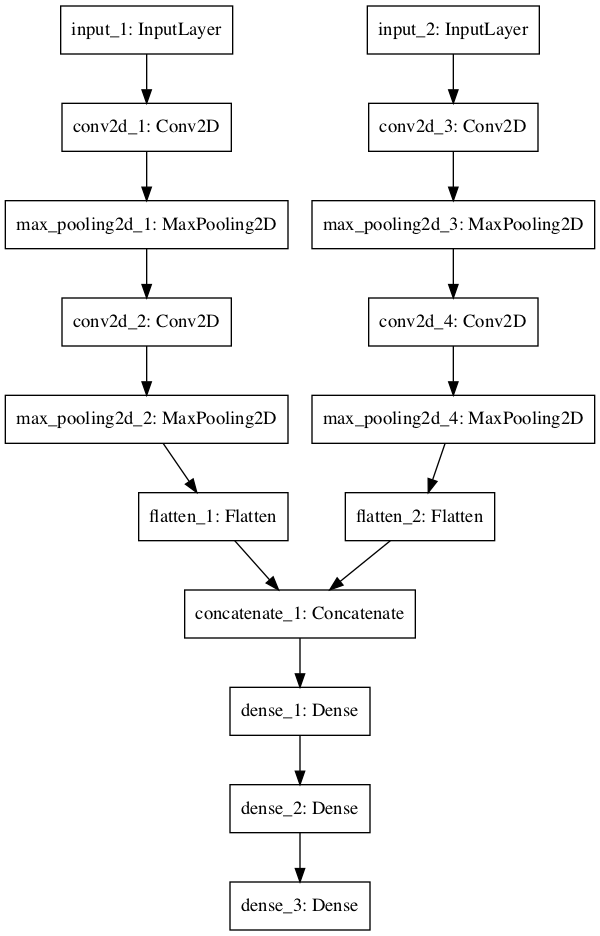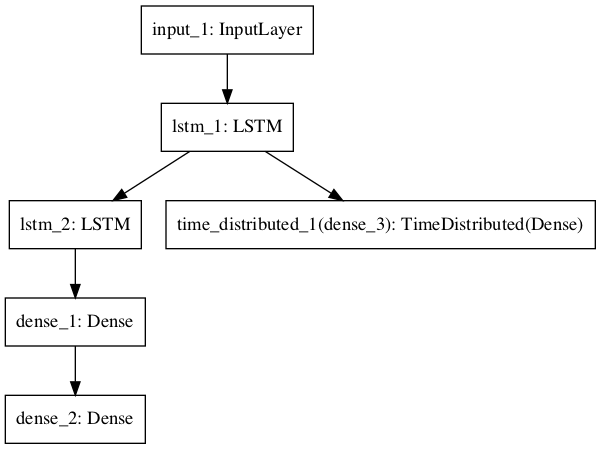# Deep Neural Frameworks

## PYTORCH

1. 1.
Deep learning with pytorch - The book
2. 2.
Pytorch DL course, git - yann lecun

1. 1.
git

## KERAS

A make sense introduction into keras, has several videos on the topic, going through many network types, creating custom activation functions, going through examples.
+ Two extra videos from the same author, examples and examples-2
1. 2.
2. 3.
Stateful LSTM - Example script showing how to use stateful RNNs to model long sequences efficiently.
3. 4.
CONV LSTM - this script demonstrate the use of a conv LSTM network, used to predict the next frame of an artificially generated move which contains moving squares.
How to force keras to use tensorflow and not teano (set the .bat file)
Batch size vs. Iterations in NN Keras.
Keras metrics - classification regression and custom metrics
Keras Metrics 2 - accuracy, ROC, AUC, classification, regression r^2.
Introduction to regression models in Keras, using MSE, comparing baseline vs wide vs deep networks.
How does Keras calculate accuracy? Formula and explanation
Compares label with the rounded predicted float, i.e. bigger than 0.5 = 1, smaller than = 0
For categorical we take the argmax for the label and the prediction and compare their location.
In both cases, we average the results.
Custom metrics (precision recall) in keras. Which are taken from here, including entropy and f1

### KERAS MULTI GPU

1. 2.
Note: probably doesn't reflect on adam, is there a reference?
2. 4.
Pitfalls in GPU training, this is a very important post, be aware that you can corrupt your weights using the wrong combination of batches-to-input-size, in keras-tensorflow. When you do multi-GPU training, it is important to feed all the GPUs with data. It can happen that the very last batch of your epoch has less data than defined (because the size of your dataset can not be divided exactly by the size of your batch). This might cause some GPUs not to receive any data during the last step. Unfortunately some Keras Layers, most notably the Batch Normalization Layer, can’t cope with that leading to nan values appearing in the weights (the running mean and variance in the BN layer).
KERAS FUNCTIONAL API
What is and how to use? A flexible way to declare layers in parallel, i.e. parallel ways to deal with input, feature extraction, models and outputs as seen in the following images.Neural Network Graph With Multiple Outputs

### Keras: Predict vs Evaluate

here:
.predict() generates output predictions based on the input you pass it (for example, the predicted characters in the MNIST example)
.evaluate() computes the loss based on the input you pass it, along with any other metrics that you requested in the metrics param when you compiled your model (such as accuracy in the MNIST example)
Keras metrics

### LOSS IN KERAS

Why is the training loss much higher than the testing loss? A Keras model has two modes: training and testing. Regularization mechanisms, such as Dropout and L1/L2 weight regularization, are turned off at testing time.
The training loss is the average of the losses over each batch of training data. Because your model is changing over time, the loss over the first batches of an epoch is generally higher than over the last batches. On the other hand, the testing loss for an epoch is computed using the model as it is at the end of the epoch, resulting in a lower loss.